# Tag: noncommutativeRaymond Smullyan‘s logic puzzles are legendary. Among his best known are his Knights (who always tell the truth) and Knaves (who always lie) puzzles. Here’s a classic example.

“On the day of his arrival, the anthropologist Edgar Abercrombie came across three inhabitants, whom we will call $A$, $B$ and $C$. He asked $A$: “Are you a Knight or a Knave?” $A$ answered, but so indistinctly that Abercrombie could not understand what he said.

He then asked $B$: “What did he say?” $B$ replies: “He said that he is a knave.” At this point, $C$ piped up and said: “Don’t believe that; it’s a lie!”

Was $C$ a Knight or a Knave?”

If you are stumped by this, try to figure out what kind of inhabitant can say “I am a Knave”.

Some years ago, my friend and co-author Karin Cvetko-Vah wrote about a much stranger island, the island of two truths.

“The island was ruled by a queen and a king. It is important to stress that the queen was neither inferior nor superior to the king. Rather than as a married couple one should think of the queen and the king as two parallel powers, somewhat like the Queen of the Night and the King Sarastro in Mozart’s famous opera The Magic Flute. The queen and the king had their own castle each, each of them had their own court, their own advisers and servants, and most importantly each of them even had their own truth value.

On the island, a proposition p is either FALSE, Q-TRUE or K-TRUE; in each of the cases we say that p has value 0, Q or K, respectively. The queen finds the truth value Q to be superior, while the king values the most the value K. The queen and the king have their opinions on all issues, while other residents typically have their opinions on some issues but not all.”

The logic of the island of two truths is the easiest example of what Karin and I called a non-commutative frame or skew Heyting algebra (see here), a notion we then used, jointly with Jens Hemelaer, to define the notion of a non-commutative topos.If you take our general definitions, and take Q as the distinguished top-element, then the truth tables for the island of two truths are these ones (value of first term on the left, that of the second on top):

$\begin{array}{c|ccc} \wedge & 0 & Q & K \\ \hline 0 & 0 & 0 & 0 \\ Q & 0 & Q & Q \\ K & 0 & K & K \end{array} \quad \begin{array}{c|ccc} \vee & 0 & Q & K \\ \hline 0 & 0 & Q & K \\ Q & Q & Q & K \\ K & K & Q & K \end{array} \quad \begin{array}{c|ccc} \rightarrow & 0 & Q & K \\ \hline 0 & Q & Q & K \\ Q & 0 & Q & K \\ K & 0 & Q & K \end{array} \quad \begin{array}{c|c} & \neg \\ \hline 0 & Q \\ Q & 0 \\ K & 0 \end{array}$

Note that on this island the order of statements is important! That is, the truth value of $p \wedge q$ may differ from that of $q \wedge p$ (and similarly for $\vee$).

Let’s reconsider Smullyan’s puzzle at the beginning of this post, but now on an island of two truths, where every inhabitant is either of Knave, or a Q-Knight (uttering only Q-valued statements), or a K-Knight (saying only K-valued statements).

Again, can you determine what type $C$ is?

Well, if you forget about the distinction between Q- and K-valued sentences, then we’re back to classical logic (or more generally, if you divide out Green’s equivalence relation from any skew Heyting algebra you obtain an ordinary Heyting algebra), and we have seen that then $B$ must be a Knave and $C$ a Knight, so in our new setting we know that $C$ is either a Q-Knight or a K-Knight, but which of the two?

Now, $C$ claims the negation of what $B$ said, so the truth value is $\neg 0 = Q$, and therefore $C$ must be a Q-Knight.

Recall that in Karin Cvetko-Vah‘s island of two truths all sentences have a unique value which can be either $0$ (false) or one of the non-false values Q or K, and the value of combined statements is given by the truth tables above. The Queen and King both have an opinion on all statements, which may or may not coincide with the actual value of that statement. However, if the Queen assigns value $0$ to a statement, then so does the King, and conversely.

Other inhabitants of the island have only their opinion about a subset of all statements (which may be empty). Two inhabitants agree on a statement if they both have an opinion on it and assign the same value to it.

Now, each inhabitant is either loyal to the Queen or to the King (or both), meaning that they agree with the Queen (resp. King) on all statements they have an opinion of. An inhabitant loyal to the Queen is said to believe a sentence when she assigns value $Q$ to it (and symmetric for those loyal to the King), and knows the statement if she believes it and that value coincides with the actual value of that statement.

Further, if A is loyal to the Queen, then the value of the statement ‘A is loyal to the Queen’ is Q, and if A is not loyal to the Queen, then the value of the sentence ‘A is loyal to the Queen’ is $0$ (and similarly for statements about loyalty to the King).

These notions are enough for the first batch of ten puzzles in Karin’s posts

Just one example:

Show that if anybody on the island knows that A is not loyal to the Queen, then everybody that has an opinion about the sentence ‘A is loyal to the Queen’ knows that.

After these two posts, Karin decided that it was more fun to blog about the use of non-commutative frames in data analysis.

But, she once gave me a text containing many more puzzles (as well as all the answers), so perhaps I’ll share these in a follow-up post.

Supernatural numbers also appear in noncommutative geometry via James Glimm’s characterisation of a class of simple $C^*$-algebras, the UHF-algebras.

A uniformly hyperfine (or, UHF) algebra $A$ is a $C^*$-algebra that can be written as the closure, in the norm topology, of an increasing union of finite-dimensional full matrix algebras

$M_{c_1}(\mathbb{C}) \subset M_{c_2}(\mathbb{C}) \subset … \quad \subset A$

Such embedding are only possible if the matrix-sizes divide each other, that is $c_1 | c_2 | c_3 | …$, and we can assign to $A$ the supernatural number $s=\prod_i c_i$ and denote $A=A(s)$.

In his paper On a certain class of operator algebras, Glimm proved that two UHF-algebras $A(s)$ and $B(t)$ are isomorphic as $C^*$-algebras if and only if $s=t$. That is, the supernatural numbers $\mathbb{S}$ are precisely the isomorphism classes of UHF-algebras.

An important invariant, the Grothendieck group $K_0$ of $A(s)$, can be described as the additive subgroup $\mathbb{Q}(s)$ of $\mathbb{Q}$ generated by all fractions of the form $\frac{1}{n}$ where $n$ is a positive integer dividing $s$.

A “noncommutative space” is a Morita class of $C^*$-algebras, so we want to know when two $UHF$-algebras $A(s)$ and $B(t)$ are Morita-equivalent. This turns out to be the case when there are positive integers $n$ and $m$ such that $n.s = m.t$, or equivalently when the $K_0$’s $\mathbb{Q}(s)$ and $\mathbb{Q}(t)$ are isomorphic as additive subgroups of $\mathbb{Q}$.

Thus Morita-equivalence defines an equivalence relation on $\mathbb{S}$ as follows: if $s=\prod p^{s_p}$ and $t= \prod p^{t_p}$ then $s \sim t$ if and only if the following two properties are satisfied:

(1): $s_p = \infty$ iff $t_p= \infty$, and

(2): $s_p=t_p$ for all but finitely many primes $p$.

That is, we can view the equivalence classes $\mathbb{S}/\sim$ as the moduli space of noncommutative spaces associated to UHF-algebras!

Now, the equivalence relation is described in terms of isomorphism classes of additive subgroups of the rationals, which was precisely the characterisation of isomorphism classes of points in the arithmetic site, that is, the finite adèle classes

$\mathbb{S}/\sim~\simeq~\mathbb{Q}^* \backslash \mathbb{A}^f_{\mathbb{Q}} / \widehat{\mathbb{Z}}^*$

and as the induced topology of $\mathbb{A}^f_{\mathbb{Q}}$ on it is trivial, this “space” is usually thought of as a noncommutative space.

That is, $\mathbb{S}/\sim$ is a noncommutative moduli space of noncommutative spaces defined by UHF-algebras.

The finite integers form one equivalence class, corresponding to the fact that the finite dimensional UHF-algebras $M_n(\mathbb{C})$ are all Morita-equivalent to $\mathbb{C}$, or a bit more pompous, that the Brauer group $Br(\mathbb{C})$ is trivial.

Multiplication of supernaturals induces a well defined multiplication on equivalence classes, and, with that multiplication we can view $\mathbb{S}/\sim$ as the ‘Brauer-monoid’ $Br_{\infty}(\mathbb{C})$ of simple UHF-algebras…

(Btw. the photo of James Glimm above was taken by George Bergman in 1972)

## F_un Mathematics

Hardly a ‘new’ blog, but one that is getting a new life! On its old homepage you’ll find a diagonal banner stating ‘This site has moved’ and clicking on it will guide you to its new location : cage.ugent.be/~kthas/Fun.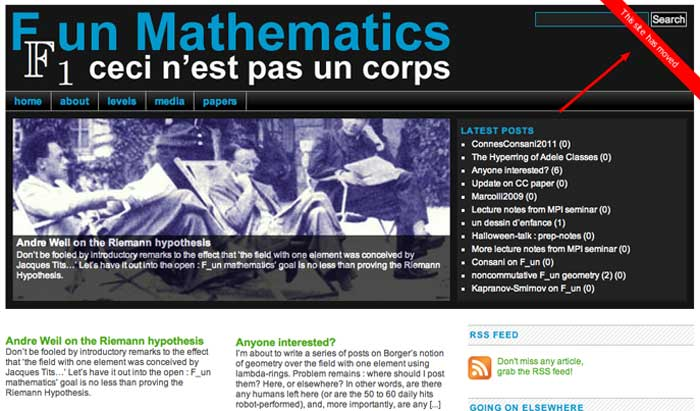From now on, this site will be hosted at the University of Ghent and maintained by Koen Thas. So, please update your bookmarks and point your RSS-aggregator to the new feed.

Everyone interested in contributing to this blog dedicated to the mathematics of the field with one element should contact Koen by email.

## angst

Though I may occasionally (cross)post at F_un mathematics, my own blog-life will center round a new blog to accompany the master-course ‘seminar noncommutative geometry’ I’m running at Antwerp University this semester. Its URL is noncommutative.org and it is called :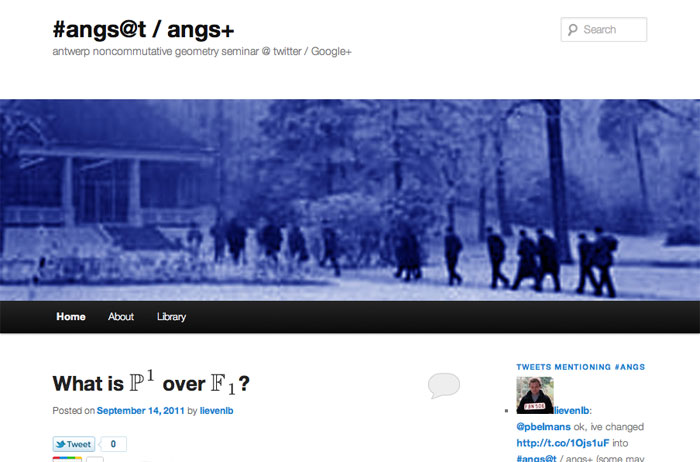Here, angs is short for Antwerp Noncommutative Geometry Seminar and the additions @t resp. + are there to indicate we will experiment a bit trying to find useful interactions between the IRL seminar, its blog and social media such as twitter and Google+.

The seminar (and blog) are scheduled to start in earnest september 30th, but I may post some prep-notes already. This semester the seminar will try to decode Smirnov’s old idea to prove the ABC-conjecture in number theory via geometry over the field with one element and connect it with new ideas such as Borger’s $\mathbb{F}_1$-geometry using $\lambda$-rings and noncommutative ideas proposed by Connes, Consani and Marcolli.

Again, anyone willing to contribute actively is invited to send me an email or to comment on ‘angst’, tweet about it using the hashtag #angs (all such tweets will appear on the frontpage) or share its posts on Google+.

## Noncommutative Arithmetic Geometry Media Library

Via the noncommutative geometry blog a new initiative maintained by Alain Connes and Katia Consani was announced : the Noncommutative Arithmetic Geometry Media Library.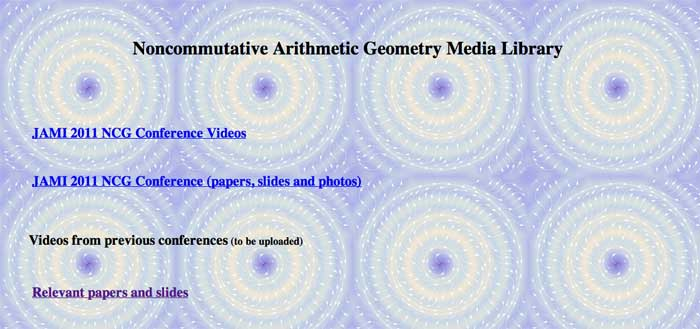This site is dedicated to maintain articles, videos, and news about meetings and activities related to noncommutative arithmetic geometry. The website is still `under construction’ and the plan is to gradually add more videos (also from past conferences and meetings), as well as papers and slides.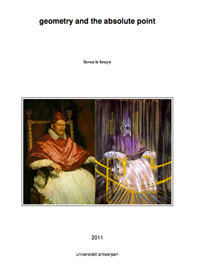In preparing for next year’s ‘seminar noncommutative geometry’ I’ve converted about 30 posts to LaTeX, centering loosely around the topics students have asked me to cover : noncommutative geometry, the absolute point (aka the field with one element), and their relation to the Riemann hypothesis.

The idea being to edit these posts thoroughly, add much more detail (and proofs) and also add some extra sections on Borger’s work and Witt rings (and possibly other stuff).

For those of you who prefer to (re)read these posts on paper or on a tablet rather than perusing this blog, you can now download the very first version (minimally edited) of the eBook ‘geometry and the absolute point’. All comments and suggestions are, of course, very welcome. I hope to post a more definite version by mid-september.

I’ve used the thesis-documentclass to keep the same look-and-feel of my other course-notes, but I would appreciate advice about turning LaTeX-files into ‘proper’ eBooks. I am aware of the fact that the memoir-class has an ebook option, and that one can use the geometry-package to control paper-sizes and margins.

Soon, I will be releasing a LaTeX-ed ‘eBook’ containing the Bourbaki-related posts. Later I might also try it on the games- and groups-related posts…

Previously, we have recalled comparisons between approaches to define a geometry over the absolute point and art-historical movements, first those due to Yuri I. Manin, subsequently some extra ones due to Javier Lopez Pena and Oliver Lorscheid.

In these comparisons, the art trend appears to have been chosen more to illustrate a key feature of the approach or an appreciation of its importance, rather than giving a visual illustration of the varieties over $\mathbb{F}_1$ the approach proposes.

Some time ago, we’ve had a couple of posts trying to depict noncommutative varieties, first the illustrations used by Shahn Majid and Matilde Marcolli, and next my own mental picture of it.

In this post, we’ll try to do something similar for affine varieties over the absolute point. To simplify things drastically, I’ll divide the islands in the Lopez Pena-Lorscheid map of $\mathbb{F}_1$ land in two subsets : the former approaches (all but the $\Lambda$-schemes) and the current approach (the $\Lambda$-scheme approach due to James Borger).

The former approaches : Francis Bacon “The Pope” (1953)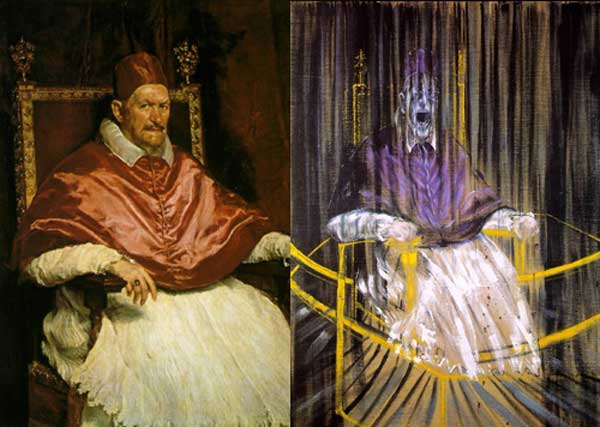The general consensus here was that in going from $\mathbb{Z}$ to $\mathbb{F}_1$ one looses the additive structure and retains only the multiplicative one. Hence, ‘commutative algebras’ over $\mathbb{F}_1$ are (commutative) monoids, and mimicking Grothendieck’s functor of points approach to algebraic geometry, a scheme over $\mathbb{F}_1$ would then correspond to a functor

$h_Z~:~\mathbf{monoids} \longrightarrow \mathbf{sets}$

Such functors are described largely by combinatorial data (see for example the recent blueprint-paper by Oliver Lorscheid), and, if the story would stop here, any Rothko painting could be used as illustration.

Most of the former approaches add something though (buzzwords include ‘Arakelov’, ‘completion at $\infty$’, ‘real place’ etc.) in order to connect the virtual geometric object over $\mathbb{F}_1$ with existing real, complex or integral schemes. For example, one can make the virtual object visible via an evaluation map $h_Z \rightarrow h_X$ which is a natural transformation, where $X$ is a complex variety with its usual functor of points $h_X$ and to connect both we associate to a monoid $M$ its complex monoid-algebra $\mathbb{C} M$. An integral scheme $Y$ can then be said to be ‘defined over $\mathbb{F}_1$’, if $h_Z$ becomes a subfunctor of its usual functor of points $h_Y$ (again, assigning to a monoid its integral monoid algebra $\mathbb{Z} M$) and $Y$ is the ‘best’ integral scheme approximation of the complex evaluation map.

To illustrate this, consider the painting Study after Velázquez’s Portrait of Pope Innocent X by Francis Bacon (right-hand painting above) which is a distorded version of the left-hand painting Portrait of Innocent X by Diego Velázquez.

Here, Velázquez’ painting plays the role of the complex variety which makes the combinatorial gadget $h_Z$ visible, and, Bacon’s painting depicts the integral scheme, build up from this combinatorial data, which approximates the evaluation map best.

All of the former approaches more or less give the same very small list of integral schemes defined over $\mathbb{F}_1$, none of them motivically interesting.

The current approach : Jackson Pollock “No. 8” (1949)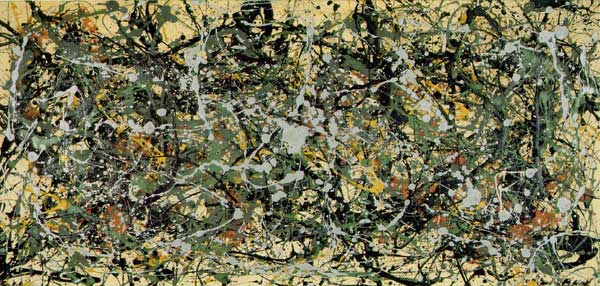An entirely different approach was proposed by James Borger in $\Lambda$-rings and the field with one element. He proposes another definition for commutative $\mathbb{F}_1$-algebras, namely $\lambda$-rings (in the sense of Grothendieck’s Riemann-Roch) and he argues that the $\lambda$-ring structure (which amounts in the sensible cases to a family of endomorphisms of the integral ring lifting the Frobenius morphisms) can be viewed as descent data from $\mathbb{Z}$ to $\mathbb{F}_1$.

The list of integral schemes of finite type with a $\lambda$-structure coincides roughly with the list of integral schemes defined over $\mathbb{F}_1$ in the other approaches, but Borger’s theory really shines in that it proposes long sought for mystery-objects such as $\mathbf{spec}(\mathbb{Z}) \times_{\mathbf{spec}(\mathbb{F}_1)} \mathbf{spec}(\mathbb{Z})$. If one accepts Borger’s premise, then this object should be the geometric object corresponding to the Witt-ring $W(\mathbb{Z})$. Recall that the role of Witt-rings in $\mathbb{F}_1$-geometry was anticipated by Manin in Cyclotomy and analytic geometry over $\mathbb{F}_1$.

But, Witt-rings and their associated Witt-spaces are huge objects, so one needs to extend arithmetic geometry drastically to include such ‘integral schemes of infinite type’. Borger has made a couple of steps in this direction in The basic geometry of Witt vectors, II: Spaces.

To depict these new infinite dimensional geometric objects I’ve chosen for Jackson Pollock‘s painting No. 8. It is no coincidence that Pollock-paintings also appeared in the depiction of noncommutative spaces. In fact, Matilde Marcolli has made the connection between $\lambda$-rings and noncommutative geometry in Cyclotomy and endomotives by showing that the Bost-Connes endomotives are universal for $\lambda$-rings.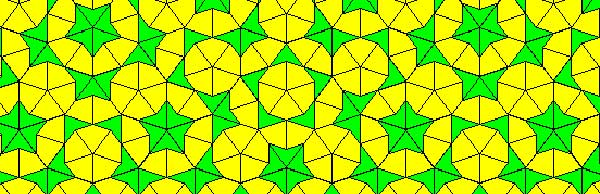Penrose tilings are aperiodic tilings of the plane, made from 2 sort of tiles : kites and darts. It is well known (see for example the standard textbook tilings and patterns section 10.5) that one can describe a Penrose tiling around a given point in the plane as an infinite sequence of 0’s and 1’s, subject to the condition that no two consecutive 1’s appear in the sequence. Conversely, any such sequence is the sequence of a Penrose tiling together with a point. Moreover, if two such sequences are eventually the same (that is, they only differ in the first so many terms) then these sequences belong to two points in the same tiling,

Another remarkable feature of Penrose tilings is their local isomorphism : fix a finite region around a point in one tiling, then in any other Penrose tiling one can find a point having an isomorphic region around it. For this reason, the space of all Penrose tilings has horrible topological properties (all points lie in each others closure) and is therefore a prime test-example for the techniques of noncommutative geometry.

In his old testament, Noncommutative Geometry, Alain Connes associates to this space a $C^*$-algebra $Fib$ (because it is constructed from the Fibonacci series $F_0,F_1,F_2,…$) which is the direct limit of sums of two full matrix-algebras $S_n$, with connecting morphisms

$S_n = M_{F_n}(\mathbb{C}) \oplus M_{F_{n-1}}(\mathbb{C}) \rightarrow S_{n+1} = M_{F_{n+1}}(\mathbb{C}) \oplus M_{F_n}(\mathbb{C}) \qquad (a,b) \mapsto ( \begin{matrix} a & 0 \\ 0 & b \end{matrix}, a)$

As such $Fib$ is an AF-algebra (for approximately finite) and hence formally smooth. That is, $Fib$ would be the coordinate ring of a smooth variety in the noncommutative sense, if only $Fib$ were finitely generated. However, $Fib$ is far from finitely generated and has other undesirable properties (at least for a noncommutative algebraic geometer) such as being simple and hence in particular $Fib$ has no finite dimensional representations…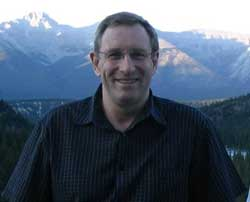A couple of weeks ago, Paul Smith discovered a surprising connection between the noncommutative space of Penrose tilings and an affine algebra in the paper The space of Penrose tilings and the non-commutative curve with homogeneous coordinate ring $\mathbb{C} \langle x,y \rangle/(y^2)$.

Giving $x$ and $y$ degree 1, the algebra $P = \mathbb{C} \langle x,y \rangle/(y^2)$ is obviously graded and noncommutative projective algebraic geometers like to associate to such algebras their ‘proj’ which is the quotient category of the category of all graded modules in which two objects become isomorphisc iff their ‘tails’ (that is forgetting the first few homogeneous components) are isomorphic.

The first type of objects NAGers try to describe are the point modules, which correspond to graded modules in which every homogeneous component is 1-dimensional, that is, they are of the form

$\mathbb{C} e_0 \oplus \mathbb{C} e_1 \oplus \mathbb{C} e_2 \oplus \cdots \oplus \mathbb{C} e_n \oplus \mathbb{C} e_{n+1} \oplus \cdots$

with $e_i$ an element of degree $i$. The reason for this is that point-modules correspond to the points of the (usual, commutative) projective variety when the affine graded algebra is commutative.

Now, assume that a Penrose tiling has been given by a sequence of 0’s and 1’s, say $(z_0,z_1,z_2,\cdots)$, then it is easy to associate to it a graded vectorspace with action given by

$x.e_i = e_{i+1}$ and $y.e_i = z_i e_{i+1}$

Because the sequence has no two consecutive ones, it is clear that this defines a graded module for the algebra $P$ and determines a point module in $\pmb{proj}(P)$. By the equivalence relation on Penrose sequences and the tails-equivalence on graded modules it follows that two sequences define the same Penrose tiling if and only if they determine the same point module in $\pmb{proj}(P)$. Phrased differently, the noncommutative space of Penrose tilings embeds in $\pmb{proj}(P)$ as a subset of the point-modules for $P$.

The only such point-module invariant under the shift-functor is the one corresponding to the 0-sequence, that is, corresponds to the cartwheel tiling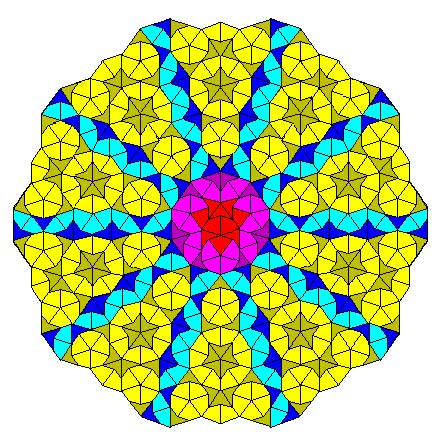Another nice consequence is that we can now explain the local isomorphism property of Penrose tilings geometrically as a consequence of the fact that the $Ext^1$ between any two such point-modules is non-zero, that is, these noncommutative points lie ‘infinitely close’ to each other.

This is the easy part of Paul’s paper.

The truly, truly amazing part is that he is able to recover Connes’ AF-algebra $Fib$ from $\pmb{proj}(P)$ as the algebra of global sections! More precisely, he proves that there is an equivalence of categories between $\pmb{proj}(P)$ and the category of all $Fib$-modules $\pmb{mod}(Fib)$!

In other words, the noncommutative projective scheme $\pmb{proj}(P)$ is actually isomorphic to an affine scheme and as its coordinate ring is formally smooth $\pmb{proj}(P)$ is a noncommutative smooth variety. It would be interesting to construct more such examples of interesting AF-algebras appearing as local rings of sections of proj-es of affine graded algebras.

The lecturers, topics and dates of the 6 mini-courses in our ‘advanced master degree 2011 in noncommutative algebra and geometry’ are :

February 21-25
Vladimir Bavula (University of Sheffield) :
Localization Theory of Rings and Modules

March 7-11
Hans-Jürgen Schneider (University of München) :
Nichols Algebra and Root Systems

April 11-12
Bernhard Keller (Université Paris VII):
Cluster Algebra and Quantum Cluster Algebras

April 18-22
Jacques Alev (Université Reims):
Automorphisms of some Basic Algebras

May 3-8
Goro Kato (Cal Poly University, San Luis Obispo, US):
Sheaf Cohomology and Zeta – Functions

May 9-13
Markus Reineke (University of Wuppertal):
Moduli Spaces of Representatives

More information can be found here. I’ve been told that some limited support is available for foreign graduate students wanting to attend this programme.Mathblogging.org is a recent initiative and may well become the default starting place to check on the status of the mathematical blogosphere.

Handy, if you want to (re)populate your RSS-aggregator with interesting mathematical blogs, is their graphical presentation of (nearly) all math-blogs ordered by type : group blogs, individual researchers, teachers and educators, journalistic writers, communities, institutions and microblogging (twitter). Links to the last 7 posts are given so you can easily determine whether that particular blog is of interest to you.

The three people behind the project, Felix Breuer, Frederik von Heymann and Peter Krautzberger, welcome you to send them links to (micro)blogs they’ve missed. Surely, there must be a lot more mathematicians with a twitter-account than the few ones listed so far…

Even more convenient is their list of latest posts from their collection, ordered by date. I’ve put that page in my Bookmarks Bar the moment I discovered it! It would be nice, if they could provide an RSS-feed of this list, so that people could place it in their sidebar, replacing old-fashioned and useless blogrolls. The site does provide two feeds, but they are completely useless as they click through to empty pages…

While we’re on the topic of math-blogging, the results of the ‘What should we write about next?’-poll that ran the previous two days on the entry page. Of all people visiting that page, 2.6% left suggestions.

The vast majority (67%) wants more posts on noncommutative geometry. Most of you are craving for introductions (and motivation) accessible to undergraduates (as ‘it’s hard to find quality, updated information on this’). In particular, you want posts giving applications in mathematics (especially number theory), or explaining relationships between different approaches. One person knew exactly how I should go about to achieve the hoped-for accessibility : “As a rule, I’d take what you think would be just right for undergrads, and then trim it down a little more.”

Others want rather specialized posts, such as on ‘connection and parallel transport in noncommutative geometry’ or on ‘trees (per J-L. Loday, M. Aguiar, Connes/Kreimer renormalization (aka Butcher group)), or something completely other tree-related’.

Fortunately, some of you told me it was fine to write about ‘combinatorial games and cool nim stuff, finite simple groups, mathematical history, number theory, arithmetic geometry’, pushed me to go for ‘anything monstrous and moonshiney’ (as if I would know the secrets of the ‘connection between the Mathieu group M24 and the elliptic genus of K3’…) or wrote that ‘various algebraic geometry related posts are always welcome: posts like Mumford’s treasure map‘.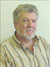Guest post by Fred Van Oystaeyen.

In my book “Virtual Topology and Functorial Geometry” (Taylor and Francis, 2009) I proposed a noncommutative version of space-time ; it is a toy model, but mathematically correct and I included a few philosophical remarks about : “What if reality is noncommutative ?”.

I want to add a few ideas about how “strange” ideas in quantum mechanics all fit naturally in the noncommutative world. First let us talk about noncommutative geometry in an intuitive way.

Then noncommutative space may be thought of as a set of noncommutative places but these noncommutative places need not be sets, in particular they are not sets of points. There is a noncommutative join $\vee$ and a noncommutative intersection $\wedge$, and they satify the axioms (very natural ones) of a noncommutative topology.

The non-commutativity is characterized by the existence of non $\wedge$-idempotent places, i.e. places with a nontrivial self intersection. This allows the $\wedge$ to be noncommutative. From algebraic geometric it follows that one may be interested to let $\vee$ be an abelian operation (hence defining a virtual topology) so let us assume this from hereon.

The set of $\wedge$-idempotent noncommutative-places forms the “commutative shadow” of the noncommutative space; it has operations $\vee$ and $\mathop{\wedge}\limits_{\bullet}$ which are abelian and $\sigma \mathop{\wedge}\limits_{\bullet}\tau$ may be thought of as the largest $\wedge$-idempotent smaller than $\sigma$ and $\tau$ in the partial ordering of the noncommutative space.

The $\wedge$-idempotent noncommutative places are sets in a commutative topology and these are the observable places in the noncommutative space. In the book I present a dynamic (time !) model allowing further elaboration on the noncommutative space but for now let us stick to the intuitive model and assume that space is in fact noncommutative with commutative shadow built upon our space time of physics.

In fact all observations, measurings and predictions made in physics are not about reality but about our observations of reality, so it may be an eternal fact that our observations of reality are described in our brains by commutative methods. Nevertheless we can observe effect of objects existing at noncommutative places in “neighboring” $\wedge$-idempotents sets or observable places.

First if an object exists at a noncommutative place it also exists at all subplaces (a harmless assumption not really essential for the rest). So if there is a noncommutative place, where some object exists, parts of this object may be observed at idempotent subplaces of the noncommutative place. These may even be disjoint in the commutative shadow, not “too far apart” as one object exists on the total noncommutative space.

Since only a part of the noncommutative object is observed on the $\wedge$-idempotent subplace it is not clear that one may actually recognize the observations at different commutative places as belonging to the same noncommutative object. Once one observes one observable place that object seems to exist only on that (commutative) place. Hence a quantum particle can be thought of as existing on several “places” but once observed it looks like it only exists there. This is a first natural phenomenon reflecting “strange” quantum mechanical principles.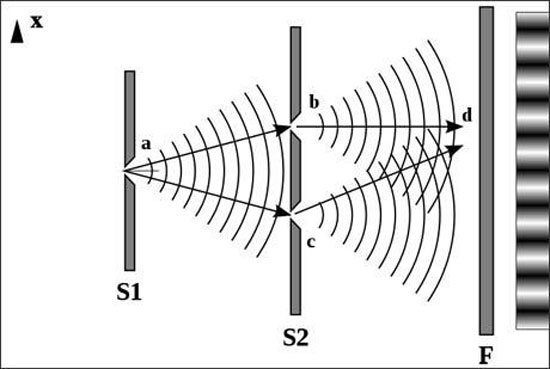Secondly let us look at the double slit experiment. The slits correspond to commutative places $\sigma_1$ and $\sigma_2$ and $\sigma_1 \mathop{\wedge}\limits_{\bullet}\sigma_2=\emptyset$, however in the noncommutative world $\sigma_1\wedge\sigma_2$ need not be empty, only it has no $\wedge$-idempotent subplaces !

Therefore if a photon is defined on a noncommutative place with “light”-effect on observable places “near enough” to it (in a neighborhood small enough to have an observable effect say) then the photon may pass though both slits without splitting or without splitting reality (parallel universes) but just moving into the noncommutative space inside $\sigma_1$ and $\sigma_2$ !

The observable effect at the slits will appear in commutative places near enough (for example, intersecting) to $\sigma_1$ or to $\sigma_2$. As the photon moves on, observable effects will appear in commutative places intersecting the one near to $\sigma_1$ or the one near to $\sigma_2$ and these may themselves have nonempty intersections.

At the moment the effect via $\sigma_1$ interacts with the effect via $\sigma_2$. As the photon progresses in its observed direction other $\wedge$-idempotents showing observable effects may meet and so several interactions between observable effects (via $\sigma_1$ and $\sigma_2$) build a picture of interference.

The symmetry of this picture actually suggests a symmetric arrangement of commutative places around a noncommutative place. So the noncommutativity of space may explain this phenomenon without holographic principle or parallel universes.

In a similar way dark mass may well be mass existing in a non-observable noncommutative place (i.e. containing no observable places). If a lot of mass is in a non-observable noncommutative place its gravity may pull matter from surrounding observable places into the noncommutative place and this may explain black holes. All kinds of problems relating to black holes may have natural non commutative solutions, e.g. information may pass from observable places to noncommutative places and is not lost, only non-observable.

In fact is the definition of information not depending on the nature and capability of the recipient ? There are many philosophically interesting ramifications of these ideas, for example every chemical or neurochemical activity should also be placed in the noncommutative space.

In the book I mentioned how “free will” could be a noncommutative space aspect of the brain activity. I also mention a possible relations with string theory. I am not a specialist in all these things but now I reached the point that I “feel” noncommutative space is a better approximation of the reality and one should investigate it further.

In view or recents events & comments, some changes have been made or will be made shortly :

categories : Sanitized the plethora of wordpress-categories to which posts belong. At the moment there are just 5 categories : ‘stories’ and ‘web’ (for all posts with low math-content) and three categories ‘level1’, ‘level2’ and ‘level3’, loosely indicating the math-difficulty of a post.

MathJax : After years of using LatexRender and WP-Latex, we’ll change to MathJax from now on. I’ll try to convert older posts as soon as possible. (Update : did a global search and replace. ‘Most’ LaTeX works, major exceptions being matrices and xymatrix commands. I’ll try to fix those later with LatexRender.)

theme : The next couple of days, the layout of this site may change randomly as I’ll be trying out things with the Swift wordpress theme. Hopefully, this will converge to a new design by next week.

name : Neverendingbooks will be renamed to something more math-related. Clearly, the new name will depend on the topics to be covered. On the main index page a pop-up poll will appear in the lower right-hand corner after 10 seconds. Please fill in the topics you’d like us to cover (no name or email required).

This poll will close on friday 21st at 12 CET and its outcome will influence name/direction of this blog. Use it also if you have a killer newname-suggestion. Among the responses so far, a funnier one : “An intro to, or motivation for non-commutative geometry, aimed at undergraduates. As a rule, I’d take what you think would be just right for undergrads, and then trim it down a little more.”

guest-posts : If you’d like to be a guest-blogger here at irregular times, please contact me. The first guest-post will be on noncommutative topology and the interpretation of quantum physics, and will appear soon. So, stay tuned…